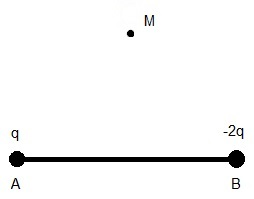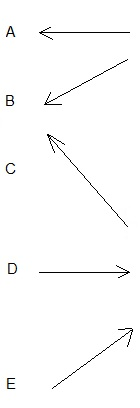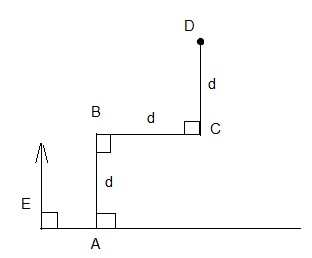# Free SAT II Physics Solutions Electric Fields and Forces

 Solutions to Free SAT II Physics Practice Questions with Solutions on Electric Fields and Forces with detailed explanations are presented. If vector F is the force between two charges q1 and q2, then what is the force between the charges 2q1 and -3q2 located at the same position as q1 and q2 respectively? A) F B) 6 F C) - F D) - 6 F E) 2 F Solution - Explanations Use Coulomb's law to write that the force F between two charges q1 and q2 is given by F = k q1 q2 / r2 u, where k is a constant, r is the distance between the charges q1 and q2 and u is a unit vector. We now substitute q1 by 2q1 and q2 by by -3q2 in the above formula to obtain the new force F2 F2 = k (2q1)(-3q2) / r2 u = -6 F Answer D. 36 J is used to move a 3 coulombs through a potential difference of V. What is V? A) 1/12 v B) 12 v C) 78 v D) 39 v E) 33 v Solution - Explanations If energy W is used to move a charge Q through a potential difference V, then W = Q V V = W / Q = 36 J / 3 C = 12 v Answer B.Fig1. - Electric Field On Perpendicular Bisector. What is the direction of the electric field at point M due to the charges q and -2q at point A and B such that M is a point on the perpendicular bisector of AB and charge q is negative?Solution - Explanations Let E1 and E2 (in red) be the electric fields due to q (negative) and -2q (positive) respectively. Since M is on the perpendicular bisector, the distance between from A to M and from B to M are equal. Therefore the magnitude of E2 is double that of E1. Hence the vector sum E1 + E2 would be directed to the left of the perpendicular bisector as shown in the figure below.Answer C.Fig3. - Work by Electric Field. What is the work done by the electric field E (assumed constant and present everywhere) to move charge q, starting from point A, along path ABCD as shown in figure 3? A) q E d B) 3 q E d C) 0 D) 2 q E d E) 4 q E d Solution - Explanations The work W1 done by the electric field E to move charge q from A to B is equal to q E d cos(θ) where θ the angle between E and AB. Hence W1 = q E d cos(0) = q E d The work W2 done by an electric field E to move charge q from B to C is equal q E d cos (θ) where θ the angle between E and BC. Hence W2 = q E d cos (π/2) = 0 The work W3 done by the electric field E to move charge q from C to D is equal to q E d cos(θ) where θ the angle between E and CD. Hence W1 = q E d cos(0) = q E d Total work = W1 + W2 + W3 = 2 q E d Answer D. The potential difference is defined as a measure of A) work done per unit charge B) Force per unit charge C) power per unit charge D) electric field per unit charge E) electric current Solution - Explanations work done per unit charge Answer A. Which of the following is correct about a closed electric circuit including a battery and a resistor? A) charges move through the circuit because the resistor supplies energy. B) charges move through the circuit because the battery maintains an electric potential difference across the ends of the external circuit. C) charges move because they are supplied by the battery. D) charges move through the circuit because they can acquire kinetic energy from the resistor. E) None of the above is correct. Solution - Explanations Charges move through the circuit because the battery maintains an electric potential difference across the ends of the external circuit. The battery supplies the electric energy needed to move charges across the circuit. Answer B. What is the work done on 5 electrons moving through a uniform electric field from a potential of 0 volts to a potential of 10 millivolts (mV). The movement is parallel to the electric field. ( charge of electron = - 1.6 � 10-19 C) A) - 8 � 10-18 J B) -1.6 10-19 J C) - 8 � 10-21 J D) 3.125 � 1017 J E) 3.125 � 1019 J Solution - Explanations The potential difference V = 10 mV - 0 = 10 mV is the work done per unit charge. Each electron has a charge of - 1.6 � 10-19 C. Hence the total work W done on the 5 electrons is given by W = 5 � 10 mV � (- 1.6 � 10-19 C); = - 8 � 10-21 J Answer C. The unit volt is defined as A) 1 joule / coulomb B) 1 coulomb / second C) 1 meter / coulomb D) 1 newton / coulomb E) 1 coulomb / joule Solution - Explanations 1 joule / coulomb Answer A. Two large metallic plates of the same size are connected each to the terminals of a battery. The distance between the plates is uniform and equal to 1 cm. What is the intensity of the electric field between the plates if the potential difference between the terminals of the battery is equal to 10 volts?(The electric field between the plates is assumed uniform) A) 10 v/m B) 0.1 v/m C) 11 v/m D) 9 v/m E)1000 v/m Solution - Explanations E = V / d = 10 / (0.01 m) = 1000 v/m Answer E. The electric field at a distance r from a charge Q is equal to E. What is the electric field at a distance 2 r from a charge 2 Q? A) E B) 2 E C) E / 2 D) 4 E E) E / 4 Solution - Explanations Electric E at a distance r due a charge Q is given by E = k Q / r2 , where k is a constant. Electric E2 at a distance 2r due a charge 2Q is given by E2 = k (2Q) / (2r) r2 = (1/2) k Q / r2 = E / 2 Answer C. Answers to the Above questions D B C D A B C A E C